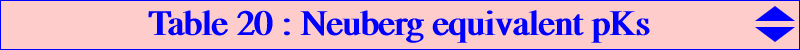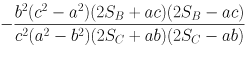When four tangents are drawn from a point M on the Neuberg cubic to the Neuberg cubic itself, their anharmonic ratio is constant (Salmon) and, up to a permutation of these tangents, is equal to :which is obtained when the tangents are those drawn from X(74) to the cubic namely AX(74), BX(74), CX(74), X(30)X(74) in this order. Now, given another pivotal cubic pK with pole Ω = p:q:r and pivot P = u:v:w, the tangents at A, B, C, P concur at P* and their anharmonic ratio is evaluated similarly. The cubic pK is said to be equivalent to the Neuberg cubic if and only if these ratios are equal. This gives the condition : ∑ a^2 (b^2 - c^2) (4SA^2 - b^2c^2) qr u^2 = 0 which can be construed as follows : • Ω lies on the circum-conic C(P) with perspector the barycentric product X526 x P^2, passing through P^2 corresponding to a degenerate pK, • P^2 lies on the trilinear polar of the barycentric quotient Ω ÷ X526 which means P lies on the diagonal conic D(Ω) with center Ω ÷ X526 passing through the four (real or not) square roots of Ω. Special cases : • with Ω = X(6), we obtain that an isogonal pK equivalent to the Neuberg cubic must have its pivot on the diagonal rectangular hyperbola passing through X(1), X(5), X(30), X(395), X(396), X(523), X(1749), etc, and whose center is the Tixier point X(476). This conic is the polar conic of the infinite point of the Euler line in the Neuberg cubic. • with Ω = X(323), D(Ω) is the Wallace hyperbola with center X(99) passing through X(1), X(2), X(20), X(63), X(147), X(194), X(487), X(488), X(616), X(617), X(627), X(628), X(1670), X(1671), X(1764), X(2128), X(2582), X(2583), X(2896), etc. • with Ω = X(50), D(Ω) is the Stammler hyperbola with center X(110) passing through X(1), X(3), X(6), X(155), X(159), X(195), X(399), X(610), X(1498), X(1740), X(2574), X(2575), X(2916), X(2917), X(2918), X(2929), X(2930), X(2931), X(2935), X(2948), X(3216), etc. • with P = X(2), C(P) passes through X(2), X(15), X(16), X(186), X(249), X(323), X(842), X(1138), X(2411), etc, giving cubics passing through the vertices of the medial triangle and whose anticomplements denoted a(pK) are also pivotal cubics with pole X(2) equivalent to K001. See light blue lines. • with P = X(1), C(P) passes through X(6), X(50), X(323), X(2436), X(2623), etc. • a pK with coincident Ω and P equivalent to K001 must have its pole/pivot on the trilinear polar of the isotomic conjugate of X526, the line passing through X(2), X(94), X(300), X(301), X(328), X(338), X(566), X(1272), X(1989), X(2410), X(2493), etc. See yellow lines. The isotomic transforms of these pKs have pivot X(2). See blue lines. *** Any projection or linear transformation, any isoconjugation with pole Q transform the Neuberg cubic into an equivalent cubic. In particular, the barycentric product of K001 by a point gives a simple equivalent cubic. The following table gives a selection of cubics pKs equivalent to the Neuberg cubic (repeated for completeness). As usual, g, t, a, c, i denote isogonal, isotomic, anticomplement, complement, inverse in (O). See also Table 68.Ω P cubic pK or centers on the cubic remark X(6) X(30) K001 X(6) X(5) K005 X(1989) X(265) K060 pK = g(K073) X(50) X(3) K073 pK = i(K001) = g(K060) X(11060) X(1989) K095 pK = X1989 x K856 X(6) X(395) K129a X(6) X(396) K129b X(11081) X(13) K261a pK = X13 x K856 X(11086) X(14) K261b pK = X14 x K856 X(76) X(3260) K276 pK = t(K001), Q = G X(1989) X(1989) K278 pK = g(K390) X(15) X(2) K341a pK = X15 x K342a X(16) X(2) K341b pK = X16 x K342b X(300) X(300) K342a pK = t(K341a) X(301) X(301) K342b pK = t(K341b) X(50) X(6) K390 pK = g(K278) X(13) X(11078) K419a pK = X13 x K867a X(14) X(11092) K419b pK = X14 x K867b X(14) X(8836) K420a X(13) X(8838) K420b X(7799) X(69) K636 pK = t(K060) X(323) X(2) K856 pK = g(K095) X(11078) X(13) K859a pK = t(K859b) X(11092) X(14) K859b pK = t(K859a) X(298) X(299) K867a pK = t(K419a) X(299) X(298) K867b pK = t(K419b) X(32) X(2173) K1042 pK = X1 x K001, Q = X(31) X(2) X(621) K1053a pK = a(K341a) X(2) X(622) K1053b pK = a(K341b) X(32) X(3129) K1054a pK = g(K1053a) X(32) X(3130) K1054b pK = g(K1053b) X(3457) X(13) K1145a pK = g(K341a) X(3458) X(14) K1145b pK = g(K341b) X(50) X(1) K1287 pK = g(K095) = X1 x K856 X(6) X(523) X(1), X(110), X(523) X(6) X(1749) X(1), X(484), X(1749) X(32) X(1953) X(6), X(19), X(48), X(1953), X(2148) pK = X1 x K005 X(50) X(195) X(54), X(195), X(1154), X(2914) X(50) X(399) X(74), X(399), X(1511), X(2914) X(323) X(63) X(63), X(2167), X(2349) X(323) X(616) X(74), X(616), X(2992) X(323) X(617) X(74), X(617), X(2993) X(323) X(627) X(54), X(627), X(1273), X(2993) X(323) X(628) X(54), X(628), X(1273), X(2992) X(393) X(1784) X(1), X(4), X(158), X(1784)Circular pKs equivalent to K001 These are the pKs corresponding to the orange cells in the table above. More generally, a circular pK with pole Ω, pivot P, isopivot Q is equivalent to K001 if and only if : • Ω lies on a circum-sextic S(Ω) passing through X(i) for i ={6, 50, 1989, 11081, 11086} and the isogonal conjugates of the Steiner ellipses infinite points. • P lies on a bicircular antigonal circum-sextic S(P) passing through X(i) for i = {3, 4, 13, 14, 30, 265, 523}. • Q lies on a bicircular inversible circum-sextic S(Q) = g S(P) passing through X(i) for i ={3, 4, 15, 16, 74, 110, 186} and the infinite points of the rectangular circum-hyperbola passing through X(186) which is the isogonal transform of the line X(3), X(125), X(131), X(265), etc.